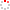NSSC OpenIR  > 空间科学部
 Bounce-averaged advection and diffusion coefficients for monochromatic electromagnetic ion cyclotron wave: Comparison between test-particle and quasi-linear models Su, Zhenpeng; Zhu, Hui; Xiao, Fuliang; Zheng, Huinan; Shen, Chao; Wang, Yuming; Wang, Shui; Su, ZP (reprint author), Univ Sci & Technol China, Dept Geophys & Planetary Sci, CAS Key Lab Geospace Environm, Hefei 230026, Anhui, Peoples R China. Department 空间科学部 Source Publication JOURNAL OF GEOPHYSICAL RESEARCH-SPACE PHYSICS2012 Volume 117Pages:A09222 ISSN 0148-0227 Language 英语 Abstract The electromagnetic ion cyclotron (EMIC) wave has long been suggested to be responsible for the rapid loss of radiation belt relativistic electrons. The test-particle simulations are performed to calculate the bounce-averaged pitch angle advection and diffusion coefficients for parallel-propagating monochromatic EMIC waves. The comparison between test-particle (TP) and quasi-linear (QL) transport coefficients is further made to quantify the influence of nonlinear processes. For typical EMIC waves, four nonlinear physical processes, i.e., the boundary reflection effect, finite perturbation effect, phase bunching and phase trapping, are found to occur sequentially from small to large equatorial pitch angles. The pitch angle averaged finite perturbation effect yields slight differences between the transport coefficients of TP and QL models. The boundary reflection effect and phase bunching produce an average reduction of >80% in the diffusion coefficients but a small change in the corresponding average advection coefficients, tending to lower the loss rate predicted by QL theory. In contrast, the phase trapping causes continuous negative advection toward the loss cone and a minor change in the corresponding diffusion coefficients, tending to increase the loss rate predicted by QL theory. For small amplitude EMIC waves, the transport coefficients grow linearly with the square of wave amplitude. As the amplitude increases, the boundary reflection effect, phase bunching and phase trapping start to occur. Consequently, the TP advection coefficients deviate from the linear growth with the square of wave amplitude, and the TP diffusion coefficients become saturated with the amplitude approaching 1 nT or above. The current results suggest that these nonlinear processes can cause significant deviation of transport coefficients from the prediction of QL theory, which should be taken into account in the future simulations of radiation belt dynamics driven by the EMIC waves.; The electromagnetic ion cyclotron (EMIC) wave has long been suggested to be responsible for the rapid loss of radiation belt relativistic electrons. The test-particle simulations are performed to calculate the bounce-averaged pitch angle advection and diffusion coefficients for parallel-propagating monochromatic EMIC waves. The comparison between test-particle (TP) and quasi-linear (QL) transport coefficients is further made to quantify the influence of nonlinear processes. For typical EMIC waves, four nonlinear physical processes, i.e., the boundary reflection effect, finite perturbation effect, phase bunching and phase trapping, are found to occur sequentially from small to large equatorial pitch angles. The pitch angle averaged finite perturbation effect yields slight differences between the transport coefficients of TP and QL models. The boundary reflection effect and phase bunching produce an average reduction of >80% in the diffusion coefficients but a small change in the corresponding average advection coefficients, tending to lower the loss rate predicted by QL theory. In contrast, the phase trapping causes continuous negative advection toward the loss cone and a minor change in the corresponding diffusion coefficients, tending to increase the loss rate predicted by QL theory. For small amplitude EMIC waves, the transport coefficients grow linearly with the square of wave amplitude. As the amplitude increases, the boundary reflection effect, phase bunching and phase trapping start to occur. Consequently, the TP advection coefficients deviate from the linear growth with the square of wave amplitude, and the TP diffusion coefficients become saturated with the amplitude approaching 1 nT or above. The current results suggest that these nonlinear processes can cause significant deviation of transport coefficients from the prediction of QL theory, which should be taken into account in the future simulations of radiation belt dynamics driven by the EMIC waves. Indexed By SCI Funding Project 中国科学院空间科学与应用研究中心 Document Type 期刊论文 Identifier http://ir.nssc.ac.cn/handle/122/3157 Collection 空间科学部 Corresponding Author Su, ZP (reprint author), Univ Sci & Technol China, Dept Geophys & Planetary Sci, CAS Key Lab Geospace Environm, Hefei 230026, Anhui, Peoples R China. Recommended CitationGB/T 7714 Su, Zhenpeng,Zhu, Hui,Xiao, Fuliang,et al. Bounce-averaged advection and diffusion coefficients for monochromatic electromagnetic ion cyclotron wave: Comparison between test-particle and quasi-linear models[J]. JOURNAL OF GEOPHYSICAL RESEARCH-SPACE PHYSICS,2012,117:A09222. APA Su, Zhenpeng.,Zhu, Hui.,Xiao, Fuliang.,Zheng, Huinan.,Shen, Chao.,...&Su, ZP .(2012).Bounce-averaged advection and diffusion coefficients for monochromatic electromagnetic ion cyclotron wave: Comparison between test-particle and quasi-linear models.JOURNAL OF GEOPHYSICAL RESEARCH-SPACE PHYSICS,117,A09222. MLA Su, Zhenpeng,et al."Bounce-averaged advection and diffusion coefficients for monochromatic electromagnetic ion cyclotron wave: Comparison between test-particle and quasi-linear models".JOURNAL OF GEOPHYSICAL RESEARCH-SPACE PHYSICS 117(2012):A09222.